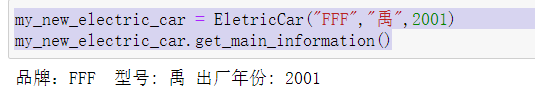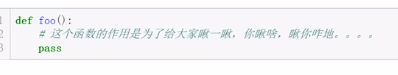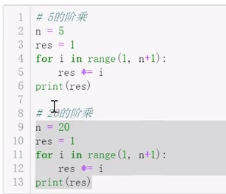+关注继续查看

# 一个简单的对象模型

Carl Friedrich Bolz 是一位在伦敦国王大学任职的研究员，他沉迷于动态语言的实现及优化等领域而不可自拔。他是 PyPy/RPython 的核心开发者之一，于此同时，他也在为 Prolog, Racket, Smalltalk, PHP 和 Ruby 等语言贡献代码。这是他的 Twitter @cfbolz 。

## 基础方法模型

 1 2 3 4 5 6 7 8 9 10 11 12 13 14 15 16 17 18 19 20 21 22 23 24 25 26 def test_read_write_field(): # Python code class A(object): pass obj = A() obj.a = 1 assert obj.a == 1 obj.b = 5 assert obj.a == 1 assert obj.b == 5 obj.a = 2 assert obj.a == 2 assert obj.b == 5 # Object model code A = Class(name="A", base_class=OBJECT, fields={}, metaclass=TYPE) obj = Instance(A) obj.write_attr("a", 1) assert obj.read_attr("a") == 1 obj.write_attr("b", 5) assert obj.read_attr("a") == 1 assert obj.read_attr("b") == 5 obj.write_attr("a", 2) assert obj.read_attr("a") == 2 assert obj.read_attr("b") == 5

 1 2 3 4 5 6 7 8 9 10 11 12 13 14 15 16 17 18 19 20 21 22 23 24 25 26 27 class Base(object): """ The base class that all of the object model classes inherit from. """ def __init__(self, cls, fields): """ Every object has a class. """ self.cls = cls self._fields = fields def read_attr(self, fieldname): """ read field 'fieldname' out of the object """ return self._read_dict(fieldname) def write_attr(self, fieldname, value): """ write field 'fieldname' into the object """ self._write_dict(fieldname, value) def isinstance(self, cls): """ return True if the object is an instance of class cls """ return self.cls.issubclass(cls) def callmethod(self, methname, *args): """ call method 'methname' with arguments 'args' on object """ meth = self.cls._read_from_class(methname) return meth(self, *args) def _read_dict(self, fieldname): """ read an field 'fieldname' out of the object's dict """ return self._fields.get(fieldname, MISSING) def _write_dict(self, fieldname, value): """ write a field 'fieldname' into the object's dict """ self._fields[fieldname] = value MISSING = object()

Base 实现了对象类的储存，同时也使用了一个字典来保存对象字段的值。现在，我们需要去实现Class 以及 Instance 类。在Instance 的构造器中将会完成类的实例化以及 fields 和 dict 初始化的操作。换句话说，Instance 只是 Base 的子类，同时并不会为其添加额外的方法。

Class 的构造器将会接受类名、基础类、类字典、以及元类这样几个操作。对于类来讲，上面几个变量都会在类初始化的时候由用户传递给构造器。同时构造器也会从它的基类那里获取变量的默认值。不过这个点，我们将在下一章节进行讲述。

 1 2 3 4 5 6 7 8 9 10 11 12 class Instance(Base): """Instance of a user-defined class. """ def __init__(self, cls): assert isinstance(cls, Class) Base.__init__(self, cls, {}) class Class(Base): """ A User-defined class. """ def __init__(self, name, base_class, fields, metaclass): Base.__init__(self, metaclass, fields) self.name = name self.base_class = base_class

 1 2 3 4 5 6 7 8 9 # set up the base hierarchy as in Python (the ObjVLisp model) # the ultimate base class is OBJECT OBJECT = Class(name="object", base_class=None, fields={}, metaclass=None) # TYPE is a subclass of OBJECT TYPE = Class(name="type", base_class=OBJECT, fields={}, metaclass=None) # TYPE is an instance of itself TYPE.cls = TYPE # OBJECT is an instance of TYPE OBJECT.cls = TYPEFigure 14.1 - Inheritance

 1 2 3 4 5 6 7 8 9 10 11 12 13 14 15 def test_read_write_field_class(): # classes are objects too # Python code class A(object): pass A.a = 1 assert A.a == 1 A.a = 6 assert A.a == 6 # Object model code A = Class(name="A", base_class=OBJECT, fields={"a": 1}, metaclass=TYPE) assert A.read_attr("a") == 1 A.write_attr("a", 5) assert A.read_attr("a") == 5

### isinstance 检查

 1 2 3 4 5 6 7 8 9 10 11 12 13 14 15 16 17 18 19 20 def test_isinstance(): # Python code class A(object): pass class B(A): pass b = B() assert isinstance(b, B) assert isinstance(b, A) assert isinstance(b, object) assert not isinstance(b, type) # Object model code A = Class(name="A", base_class=OBJECT, fields={}, metaclass=TYPE) B = Class(name="B", base_class=A, fields={}, metaclass=TYPE) b = Instance(B) assert b.isinstance(B) assert b.isinstance(A) assert b.isinstance(OBJECT) assert not b.isinstance(TYPE)

 1 2 3 4 5 6 7 8 9 10 11 12 13 class Class(Base): ... def method_resolution_order(self): """ compute the method resolution order of the class """ if self.base_class is None: return [self] else: return [self] + self.base_class.method_resolution_order() def issubclass(self, cls): """ is self a subclass of cls? """ return cls in self.method_resolution_order()

### 方法调用

 1 2 3 4 5 6 7 8 9 10 11 12 13 14 15 16 17 18 19 20 21 22 23 24 25 26 27 def test_callmethod_simple(): # Python code class A(object): def f(self): return self.x + 1 obj = A() obj.x = 1 assert obj.f() == 2 class B(A): pass obj = B() obj.x = 1 assert obj.f() == 2 # works on subclass too # Object model code def f_A(self): return self.read_attr("x") + 1 A = Class(name="A", base_class=OBJECT, fields={"f": f_A}, metaclass=TYPE) obj = Instance(A) obj.write_attr("x", 1) assert obj.callmethod("f") == 2 B = Class(name="B", base_class=A, fields={}, metaclass=TYPE) obj = Instance(B) obj.write_attr("x", 2) assert obj.callmethod("f") == 3

 1 2 3 4 5 6 7 8 class Class(Base): ... def _read_from_class(self, methname): for cls in self.method_resolution_order(): if methname in cls._fields: return cls._fields[methname] return MISSING

 1 2 3 4 5 6 7 8 9 10 11 12 13 14 15 16 17 18 19 20 21 22 23 24 25 26 27 28 29 30 def test_callmethod_subclassing_and_arguments(): # Python code class A(object): def g(self, arg): return self.x + arg obj = A() obj.x = 1 assert obj.g(4) == 5 class B(A): def g(self, arg): return self.x + arg * 2 obj = B() obj.x = 4 assert obj.g(4) == 12 # Object model code def g_A(self, arg): return self.read_attr("x") + arg A = Class(name="A", base_class=OBJECT, fields={"g": g_A}, metaclass=TYPE) obj = Instance(A) obj.write_attr("x", 1) assert obj.callmethod("g", 4) == 5 def g_B(self, arg): return self.read_attr("x") + arg * 2 B = Class(name="B", base_class=A, fields={"g": g_B}, metaclass=TYPE) obj = Instance(B) obj.write_attr("x", 4) assert obj.callmethod("g", 4) == 12

## 基础属性模型

 1 result = obj.f(arg1, arg2)

 1 2 method = obj.f result = method(arg1, arg2)

 1 2 3 4 5 6 7 8 9 10 11 12 13 14 15 16 17 18 19 20 21 22 23 24 25 26 27 28 29 30 31 def test_bound_method(): # Python code class A(object): def f(self, a): return self.x + a + 1 obj = A() obj.x = 2 m = obj.f assert m(4) == 7 class B(A): pass obj = B() obj.x = 1 m = obj.f assert m(10) == 12 # works on subclass too # Object model code def f_A(self, a): return self.read_attr("x") + a + 1 A = Class(name="A", base_class=OBJECT, fields={"f": f_A}, metaclass=TYPE) obj = Instance(A) obj.write_attr("x", 2) m = obj.read_attr("f") assert m(4) == 7 B = Class(name="B", base_class=A, fields={}, metaclass=TYPE) obj = Instance(B) obj.write_attr("x", 1) m = obj.read_attr("f") assert m(10) == 12

 1 2 3 4 5 6 7 8 9 10 11 12 13 14 15 16 17 18 19 20 21 22 23 24 25 26 class Base(object): ... def read_attr(self, fieldname): """ read field 'fieldname' out of the object """ result = self._read_dict(fieldname) if result is not MISSING: return result result = self.cls._read_from_class(fieldname) if _is_bindable(result): return _make_boundmethod(result, self) if result is not MISSING: return result raise AttributeError(fieldname) def callmethod(self, methname, *args): """ call method 'methname' with arguments 'args' on object """ meth = self.read_attr(methname) return meth(*args) def _is_bindable(meth): return callable(meth) def _make_boundmethod(meth, self): def bound(*args): return meth(self, *args) return bound

## 元对象协议

### 自定义属性读写操作

__getattr__ 方法将会在属性通过常规方法无法查找到的情况下被调用，换句话说，在实例字典、类字典、父类字典等等对象中都找不到对应的属性时，会触发该方法的调用。我们将传入一个被查找属性的名字作为这个方法的参数。在早期的 Smalltalk4 中这个方法被称为 doesNotUnderstand: 。

 1 2 3 4 5 6 7 8 9 10 11 12 13 14 15 16 17 18 19 20 21 22 23 24 25 26 27 28 29 30 31 32 33 34 35 36 37 38 39 40 41 42 43 44 45 46 47 def test_getattr(): # Python code class A(object): def __getattr__(self, name): if name == "fahrenheit": return self.celsius * 9\. / 5\. + 32 raise AttributeError(name) def __setattr__(self, name, value): if name == "fahrenheit": self.celsius = (value - 32) * 5\. / 9. else: # call the base implementation object.__setattr__(self, name, value) obj = A() obj.celsius = 30 assert obj.fahrenheit == 86 # test __getattr__ obj.celsius = 40 assert obj.fahrenheit == 104 obj.fahrenheit = 86 # test __setattr__ assert obj.celsius == 30 assert obj.fahrenheit == 86 # Object model code def __getattr__(self, name): if name == "fahrenheit": return self.read_attr("celsius") * 9\. / 5\. + 32 raise AttributeError(name) def __setattr__(self, name, value): if name == "fahrenheit": self.write_attr("celsius", (value - 32) * 5\. / 9.) else: # call the base implementation OBJECT.read_attr("__setattr__")(self, name, value) A = Class(name="A", base_class=OBJECT, fields={"__getattr__": __getattr__, "__setattr__": __setattr__}, metaclass=TYPE) obj = Instance(A) obj.write_attr("celsius", 30) assert obj.read_attr("fahrenheit") == 86 # test __getattr__ obj.write_attr("celsius", 40) assert obj.read_attr("fahrenheit") == 104 obj.write_attr("fahrenheit", 86) # test __setattr__ assert obj.read_attr("celsius") == 30 assert obj.read_attr("fahrenheit") == 86

 1 2 3 4 5 6 7 8 9 10 11 12 13 14 15 16 17 18 19 20 21 22 class Base(object): ... def read_attr(self, fieldname): """ read field 'fieldname' out of the object """ result = self._read_dict(fieldname) if result is not MISSING: return result result = self.cls._read_from_class(fieldname) if _is_bindable(result): return _make_boundmethod(result, self) if result is not MISSING: return result meth = self.cls._read_from_class("__getattr__") if meth is not MISSING: return meth(self, fieldname) raise AttributeError(fieldname) def write_attr(self, fieldname, value): """ write field 'fieldname' into the object """ meth = self.cls._read_from_class("__setattr__") return meth(self, fieldname, value)

 1 2 3 def OBJECT__setattr__(self, fieldname, value): self._write_dict(fieldname, value) OBJECT = Class("object", None, {"__setattr__": OBJECT__setattr__}, None)

OBJECT.__setattr__ 的具体实现和之前 write_attr 方法的实现有着相似之处。在完成这些修改后，我们可以顺利的通过我们的测试。

### 描述符协议

 1 2 3 4 5 6 7 8 9 10 11 12 13 14 15 16 17 18 19 20 21 22 23 def test_get(): # Python code class FahrenheitGetter(object): def __get__(self, inst, cls): return inst.celsius * 9\. / 5\. + 32 class A(object): fahrenheit = FahrenheitGetter() obj = A() obj.celsius = 30 assert obj.fahrenheit == 86 # Object model code class FahrenheitGetter(object): def __get__(self, inst, cls): return inst.read_attr("celsius") * 9\. / 5\. + 32 A = Class(name="A", base_class=OBJECT, fields={"fahrenheit": FahrenheitGetter()}, metaclass=TYPE) obj = Instance(A) obj.write_attr("celsius", 30) assert obj.read_attr("fahrenheit") == 86

__get__ 方法将会在属性查找完后被 FahrenheitGetter 实例所调用。传递给 __get__ 的参数是查找过程结束时所处的那个实例。

 1 2 3 4 5 def _is_bindable(meth): return hasattr(meth, "__get__") def _make_boundmethod(meth, self): return meth.__get__(self, None)

## 实例优化

Map 优化利用了这样一个事实。它将会将每个实例的字典分割为两个部分。一部分存放可以在所有实例中共享的属性名。然后另一部分只存放对第一部分产生的 Map 的引用和存放具体的值。存放属性名的map 将会作为值的索引。

 1 2 3 4 5 6 7 8 9 10 11 12 13 14 15 16 17 18 19 20 21 22 23 24 25 def test_maps(): # white box test inspecting the implementation Point = Class(name="Point", base_class=OBJECT, fields={}, metaclass=TYPE) p1 = Instance(Point) p1.write_attr("x", 1) p1.write_attr("y", 2) assert p1.storage == [1, 2] assert p1.map.attrs == {"x": 0, "y": 1} p2 = Instance(Point) p2.write_attr("x", 5) p2.write_attr("y", 6) assert p1.map is p2.map assert p2.storage == [5, 6] p1.write_attr("x", -1) p1.write_attr("y", -2) assert p1.map is p2.map assert p1.storage == [-1, -2] p3 = Instance(Point) p3.write_attr("x", 100) p3.write_attr("z", -343) assert p3.map is not p1.map assert p3.map.attrs == {"x": 0, "z": 1}

p1 的包含 attrs 的 map 存放了 x 和 y 两个属性，其在 p1 中存放的值分别为 0 和 1。然后创建第二个实例 p2 ，并通过同样的方法网同样的 map 中添加同样的属性。 换句话说，如果不同的属性被添加了，那么其中的 map 是不通用的。

Map 类长下面这样：

 1 2 3 4 5 6 7 8 9 10 11 12 13 14 15 16 17 18 class Map(object): def __init__(self, attrs): self.attrs = attrs self.next_maps = {} def get_index(self, fieldname): return self.attrs.get(fieldname, -1) def next_map(self, fieldname): assert fieldname not in self.attrs if fieldname in self.next_maps: return self.next_maps[fieldname] attrs = self.attrs.copy() attrs[fieldname] = len(attrs) result = self.next_maps[fieldname] = Map(attrs) return result EMPTY_MAP = Map({})

Map 类拥有两个方法，分别是 get_index 和 next_map 。前者用于查找对象储存空间中的索引中查找对应的属性名称。而在新的属性添加到对象中时应该使用后者。在这种情况下，不同的实例需要用next_map 计算不同的映射关系。这个方法将会使用 next_maps 来查找已经存在的映射。这样，相似的实例将会使用相似的 Map 对象。Figure 14.2 - Map transitions

 1 2 3 4 5 6 7 8 9 10 11 12 13 14 15 16 17 18 19 20 21 22 23 class Instance(Base): """Instance of a user-defined class. """ def __init__(self, cls): assert isinstance(cls, Class) Base.__init__(self, cls, None) self.map = EMPTY_MAP self.storage = [] def _read_dict(self, fieldname): index = self.map.get_index(fieldname) if index == -1: return MISSING return self.storage[index] def _write_dict(self, fieldname, value): index = self.map.get_index(fieldname) if index != -1: self.storage[index] = value else: new_map = self.map.next_map(fieldname) self.storage.append(value) self.map = new_map

## 潜在扩展

• 最简单的是添加更多的特殊方法方法，比如一些 __init____getattribute____set__ 这样非常容易实现和有趣的方法。

• 扩展模型支持多重继承。为了实现这一点，每一个类都需要一个父类列表。然后Class.method_resolution_order 需要进行修改，以便支持方法查找。一个简单的 MRO 计算规则可以使用深度优先原则。然后更为复杂的可以采用C3 算法, 这种算法能更好的处理菱形继承结构所带来的一些问题。

• 一个更为疯狂的想法是切换到原型模式，这需要消除类和实例之间的差别。

# 参考文献

1. P. Cointe, “Metaclasses are first class: The ObjVlisp Model,” SIGPLAN Not, vol. 22, no. 12, pp. 156–162, 1987.↩

2. It seems that the attribute-based model is conceptually more complex, because it needs both method lookup and call. In practice, calling something is defined by looking up and calling a special attribute __call__, so conceptual simplicity is regained. This won’t be implemented in this chapter, however.)↩

3. G. Kiczales, J. des Rivieres, and D. G. Bobrow, The Art of the Metaobject Protocol. Cambridge, Mass: The MIT Press, 1991.↩

4. A. Goldberg, Smalltalk-80: The Language and its Implementation. Addison-Wesley, 1983, page 61.↩

5. In Python the second argument is the class where the attribute was found, though we will ignore that here.↩

6. C. Chambers, D. Ungar, and E. Lee, “An efficient implementation of SELF, a dynamically-typed object-oriented language based on prototypes,” in OOPSLA, 1989, vol. 24.↩

7. How that works is beyond the scope of this chapter. I tried to give a reasonably readable account of it in a paper I wrote a few years ago. It uses an object model that is basically a variant of the one in this chapter: C. F. Bolz, A. Cuni, M. Fijałkowski, M. Leuschel, S. Pedroni, and A. Rigo, “Runtime feedback in a meta-tracing JIT for efficient dynamic languages,” in Proceedings of the 6th Workshop on Implementation, Compilation, Optimization of Object-Oriented Languages, Programs and Systems, New York, NY, USA, 2011, pp. 9:1–9:8.↩0 00 00 00 0Python编程：MySQLdb模块对数据库的基本增删改查操作
Python编程：MySQLdb模块对数据库的基本增删改查操作
0 0Python编程：entry_points将Python模块转变为命令行工具
Python编程：entry_points将Python模块转变为命令行工具
0 0Python编程：SQLAlchemy查询数据没有变化

0 0Python编程：运行目录或zip压缩文件
Python编程：运行目录或zip压缩文件
0 0Python编程：PyThink数据库交互模块提高爬虫编写速度
Python编程：PyThink数据库交互模块提高爬虫编写速度
0 0Python编程：glob模块进行文件名模式匹配
Python编程：glob模块进行文件名模式匹配
0 0

Python常用数据科学库136253

Python Web开发基础329884

【Python入门】Python数据处理编程基础2726

Python Web 框架 Django 快速入门302564

Python 脚本入门197283046531